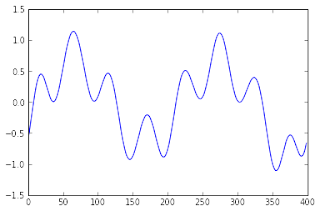### Signal Filtering using inverse FFT in Python

A straight forward way of doing signal filtering is zeroing out terms in inverse FFT result. I saw a good post online. However, it does not encapsulate into a function nor allow users to specify passing bands in terms of physical frequency. So I modified it into a function below.


def bandpass_ifft(X, Low_cutoff, High_cutoff, F_sample, M=None):
"""Bandpass filtering on a real signal using inverse FFT

Inputs
=======

X: 1-D numpy array of floats, the real time domain signal (time series) to be filtered
Low_cutoff: float, frequency components below this frequency will not pass the filter (physical frequency in unit of Hz)
High_cutoff: float, frequency components above this frequency will not pass the filter (physical frequency in unit of Hz)
F_sample: float, the sampling frequency of the signal (physical frequency in unit of Hz)

Notes
=====
1. The input signal must be real, not imaginary nor complex
2. The Filtered_signal will have only half of original amplitude. Use abs() to restore.
3. In Numpy/Scipy, the frequencies goes from 0 to F_sample/2 and then from negative F_sample to 0.

"""

import scipy, numpy
if M == None: # if the number of points for FFT is not specified
M = X.size # let M be the length of the time series
Spectrum = scipy.fft(X, n=M)
[Low_cutoff, High_cutoff, F_sample] = map(float, [Low_cutoff, High_cutoff, F_sample])

#Convert cutoff frequencies into points on spectrum
[Low_point, High_point] = map(lambda F: F/F_sample * M /2, [Low_cutoff, High_cutoff])# the division by 2 is because the spectrum is symmetric

Filtered_spectrum = [Spectrum[i] if i >= Low_point and i <= High_point else 0.0 for i in xrange(M)] # Filtering
Filtered_signal = scipy.ifft(Filtered_spectrum, n=M)  # Construct filtered signal
return Spectrum, Filtered_spectrum, Filtered_signal, Low_point, High_point


Here is an example showing its usage.

First of all, let's generate a signal of different frequencies.


import numpy
N = 400 # signal length of number
x = numpy.arange(0, N, 1) # generate the time ticks
Sines = [numpy.sin(x*n)*(1-n) for n in [.9, .75, .5, .25, .12, .03, 0.025]]# different frequency components
y = numpy.sum(Sines, axis=0)   # add them by column, low frequencies have higher amplitudes

import matplotlib.pyplot as plt
plt.plot(x, y) # visualize the data


The raw signal looks like this:Then, let's assume the sampling frequency is 500Hz and we only want signals between 5 to 30 Hz to go thru the filter.


Low_cutoff, High_cutoff, F_sample = 5, 30, 500
Spectrum, Filtered_spectrum, Filtered_signal, Low_point, High_point = bandpass_ifft(y, Low_cutoff, High_cutoff, F_sample)

# Below is visualization
fig1 = plt.figure()
plt.stem(numpy.fft.fftfreq(N)*F_sample, abs(Spectrum))
plt.axis([0, F_sample/2, None, None]) # since the signal is real, the spectrum is symmetric|
plt.axvspan(Low_cutoff, High_cutoff,fc='g',alpha='.5') # The frequencies that we wanna get rid of

fig2 = plt.figure()
plt.plot(x, Filtered_signal)


In the first figure plotted, the green box shows frequencies that will be kept by the filter.Here is the filtered signal. As you can see, high frequencies are gone. The signal looks very smooth.Of course, this approach is very costly: FFT and iFFT are both $O(n\lg n)$ complexity algorithms. It is much more costly than convolving signal with filter terms (what people normally do in digital signal processing). Oh, and it cannot be done in real-time - you must wait until all data is sampled.

There is also a minor implementation issue here. Both Scipy and Numpy provides FFT/iFFT functions. But their speeds vary . If you wanna go faster, try Python binding to some C/C++-based FFT libraries.

References:
1. http://stackoverflow.com/questions/6363154/what-is-the-difference-between-numpy-fft-and-scipy-fftpack

#### 1 comment:

ice_lin said...

nice work, but it seems that this code set the all the power in [negative F_sample, 0] to zero, including the corresponding power btw [Low_cutoff, High_cutoff]. Doesn't this make some power loss in ifft?